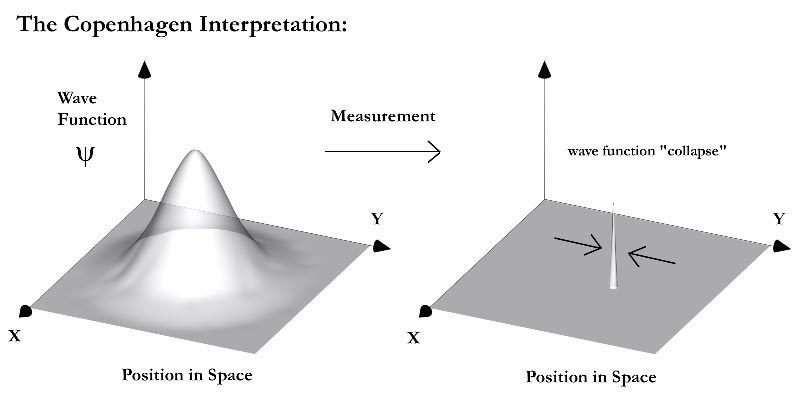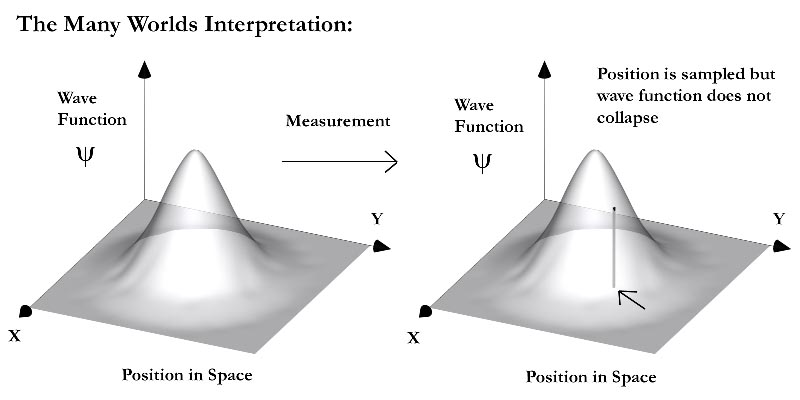In my article I had a bunch of sidebars discussing the theoretical motivations for believing in multiple universes. One such motivation comes from what I believe to be the most compelling interpretation of one of our foremost physical theories, quantum mechanics. The two major interpretations of quantum mechanics are called the Copenhagen and Many Worlds Interpretations. These graphics are meant to capture the essence of each interpretation. In Quantum Mechanics, the central object is what physicists call the "wave function", a mathematical object whose square tells you the probability that a particle will be found at a particular place in space and time. Mathematically, the wave function is the solution to the famous Schrödinger Equation, the key equation of quantum mechanics. In the standard interpretation, one can think of the wave function itself as something like a Gaussian Bell Curve that keeps its shape until a measurement is made, whereupon the bell curve gets squished down to a spike at the position of the particle. This is what physicists term the "collapse" of the wave function, and the idea of wave function collapse forms the backbone of the standard interpretation of quantum mechanics, the Copenhagen interpretation. The problem is that there is no known physical mechanism that explains when and how the wave function should collapse, and in addition, wave function collapse violates the Schrödinger Equation and leads to the philosophical interpretation that objects do not exist until we observe them. Knowing this, in 1957, Hugh Everett proposed a much simpler interpretation that simply says, the Schrödinger Equation is obeyed at all times and the wave function never collapses. This means that when a measurement is made, we are not limited to a single outcome, or "spike", but in fact, all possible outcomes from the entire Bell Curve are realized somewhere. Since these other "somewheres" are quite clearly other universes, Everett's interpretation garnered the name The Many Worlds Interpretation of Quantum Mechanics.In the Copenhagen Interpretation, a measurement collapses the wave function (shown here as a 3D Gaussian bell curve) to a spike at some position and the particle is said to exist at that point and nowhere else, to a precision limited by the famous Heisenberg Uncertainty Principle.In the Many Worlds Interpretation, measurement does not collapse the wave function, it merely samples one possible position from the probability distribution. All other possible measurements are also physically actualized somewhere, i.e. in other universes in the Multiverse.